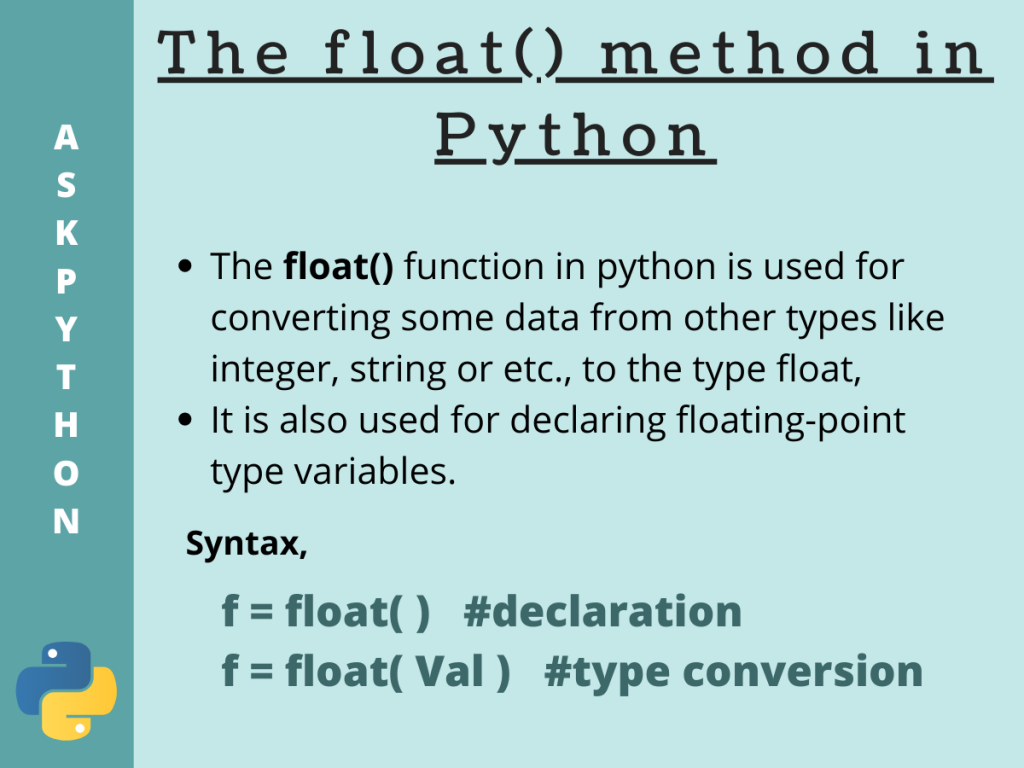# The Python float() Method## Introduction

All Python features are associated with objects. Python float creates floating-point objects in Python. It is one of the primitive elements of Python programming.

Further all kinds of objects are classified into types. Some of the basic native types in python are strings, integer, complex numbers and finally floating-point numbers.

Focusing on the floating-point type, the native Python `float()` method is used to create a float type object as well as to type-cast any other type to the corresponding float.

So, in this tutorial, we are going to discuss what the Python `float()` method does, as well as how it can be used in our code accordingly.

## Python float() basics

Basically, the Python float() function is used for converting some data from other types like integer, string or etc., to the type float. It is also used for declaring floating-point type variables.

Let us now see how the function looks like,

```f = float( )
```

Here, `f` is the new object of type float. We can also convert from other types too. Let us see how

```f = float( Val )
```

Now here, `Val` is the data of some other type which gets type-casted to float and is then stored inside the `f` variable( float type ).

## Python float() Method Usage

Take a look at the example below. Here we try to calculate the area of a rectangle with user-defined length and breadth.

```#taking the length and breadth of the rectangle as user inputs
len=input("Enter the length of the rectangle : ")
brdth=input("Enter the breadth of the rectangle : ")

#at this point both len and brdth are holding str values

area=float() #area declared as float() object

#len and brdth type-casted to float for * operation
area=float(len)*float(brdth)
#area is calculated
print("The area of the given rectangle is : ",area)
print("type of area= ",type(area))
```

Output:

In this code above,

• At first, we take the length as well as the breadth of the rectangle as user inputs. Which by default is stored as string type values
• Then we declare a variable `area`, an object of float type, which is going to store the area of the rectangle in future
• After that, we explicitly convert the string type `len` and `brdth` parameters into floating-point type using the `float()` method. And further calculate the area i.e., len*brdth, and print the same
• Finally, we print the `type()` of area, which as expected turns out as float type itself

## Various uses of Python float()

Other than the object declaration, the `float()` method can also be used for converting other data types to float type and also used with some special parameters. Let us see how the function works according to the parameter we pass.

### 1. Using float() for type-casting

We already saw that we can explicitly convert any variable of any data type into float type in the above example. For better understanding let us see how the method works for different data types like strings, and integers.

```s=100
print("s=",s)
print("Type before : ",type(s))
s=float(s)
print("s=",s)
print("Type after : ",type(s))
```

Output:

```s= 100
Type before :  <class 'int'>
s= 100.0
Type after :  <class 'float'>
```

Here, as we can observe, the type of the variable is converted from integer to floating type.

Previously we saw the type conversion of string into float at the beginning. In that case, the strings `len` and `brdth` were numbers. What if the string is not any number? Let us see,

```s="AskPython"
print("s=",s)
print("Type before : ",type(s))
s=float(s)
print("s=",s)
print("Type after : ",type(s))
```

Output:

```s= AskPython
Type before :  <class 'str'>
Traceback (most recent call last):
File "C:/Users/sneha/Desktop/test.py", line 4, in <module>
s=float(s)
ValueError: could not convert string to float: 'AskPython'
```

From this, we can infer that the `float()` method only can convert a value of string type into a floating-point number. And throws a ValueError if the string contains any character in it.

### 2. Using float() with Special parameters

What is going to happen if we pass nothing, infinity, or NaN(Not a Number) as parameters to the `float()` method? Would it be able to convert them into float? Let us see what happens in the example below,

```val1=float('inf')
val2=float('NaN')
val=float()
print("Default value of float()= ",val,";Type-",type(val))
print("float('inf') = ",val1,";Type-",type(val))
print("float('NaN')= ",val2,";Type-",type(val))
```

Output:

• passing nothing to the `float()` method gives us its default value, which in fact is ‘0.0’,
• for inf or infinity, the returned value was also `inf`, but with a type converted to float,
• Similarly, for NaN, the function returns `nan` with the type float.
So, in this tutorial, we learned about the` float()` method in Python, how it is used and what the function does. For any further questions, comments are always welcome.